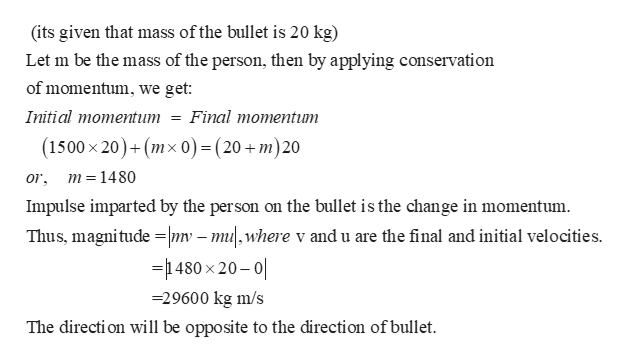# A bullet (mass of 20 kg) is fired at a person wearing a bulletproof vest at 1500 m/s. The person is knocked back at 20 m/s but survives. The bullet just stops.a. What are the magnitude and direction of the impulse imparted by the person on the bullet?b. Calculate the force exerted by the person on the bullet (ignore gravity).

Question
40 views

A bullet (mass of 20 kg) is fired at a person wearing a bulletproof vest at 1500 m/s. The person is knocked back at 20 m/s but survives. The bullet just stops.

a. What are the magnitude and direction of the impulse imparted by the person on the bullet?

b. Calculate the force exerted by the person on the bullet (ignore gravity).

check_circle

Step 1

(a) The magnitude and direction of the impulse...help_outlineImage Transcriptionclose(its given that mass ofthe bullet is 20 kg) Let m be the mass of the person, then by applying conservation of momentum, we get Initial momentum = Final momentum (1500 x 20)+(mx o) =(20+ m)20 m =1480 or Impulse imparted by the person on the bullet is the change in momentum Thus, magnitude nv -mu,where v and u are the final and initial velocities =480 x 20-0 29600 kg m/s The directi on will be opposite to the direction of bullet fullscreen

### Want to see the full answer?

See Solution

#### Want to see this answer and more?

Solutions are written by subject experts who are available 24/7. Questions are typically answered within 1 hour.*

See Solution
*Response times may vary by subject and question.
Tagged in

### Other